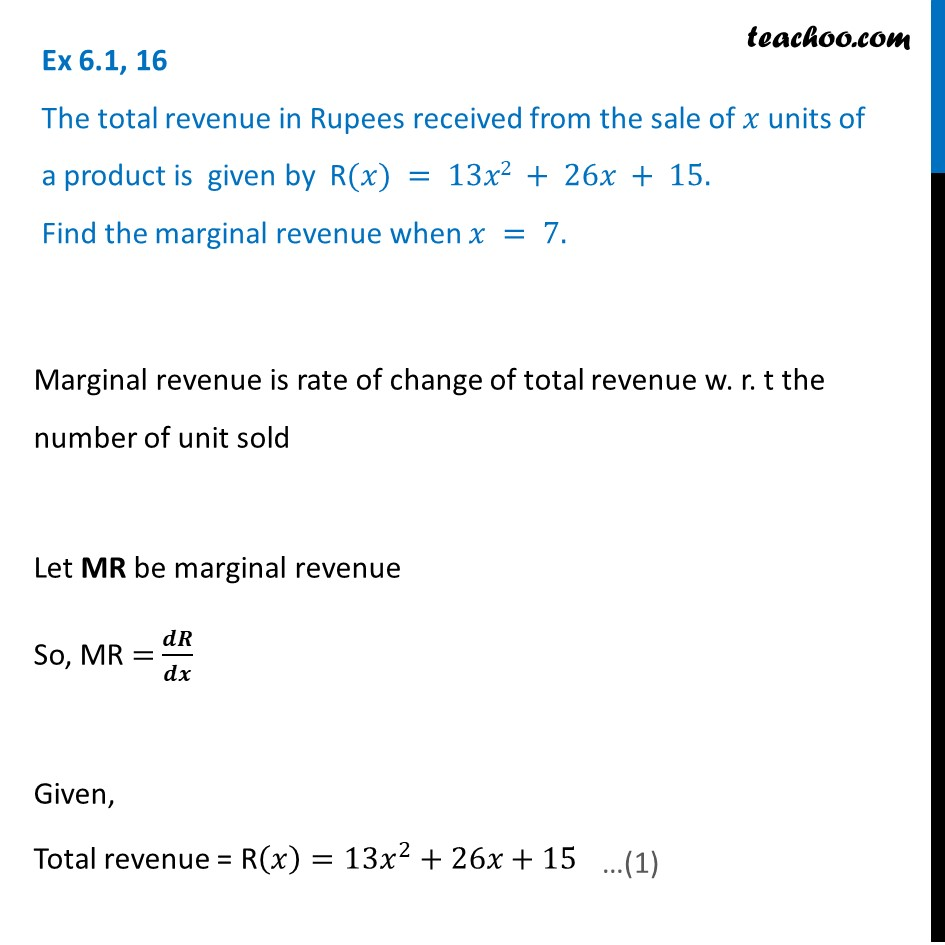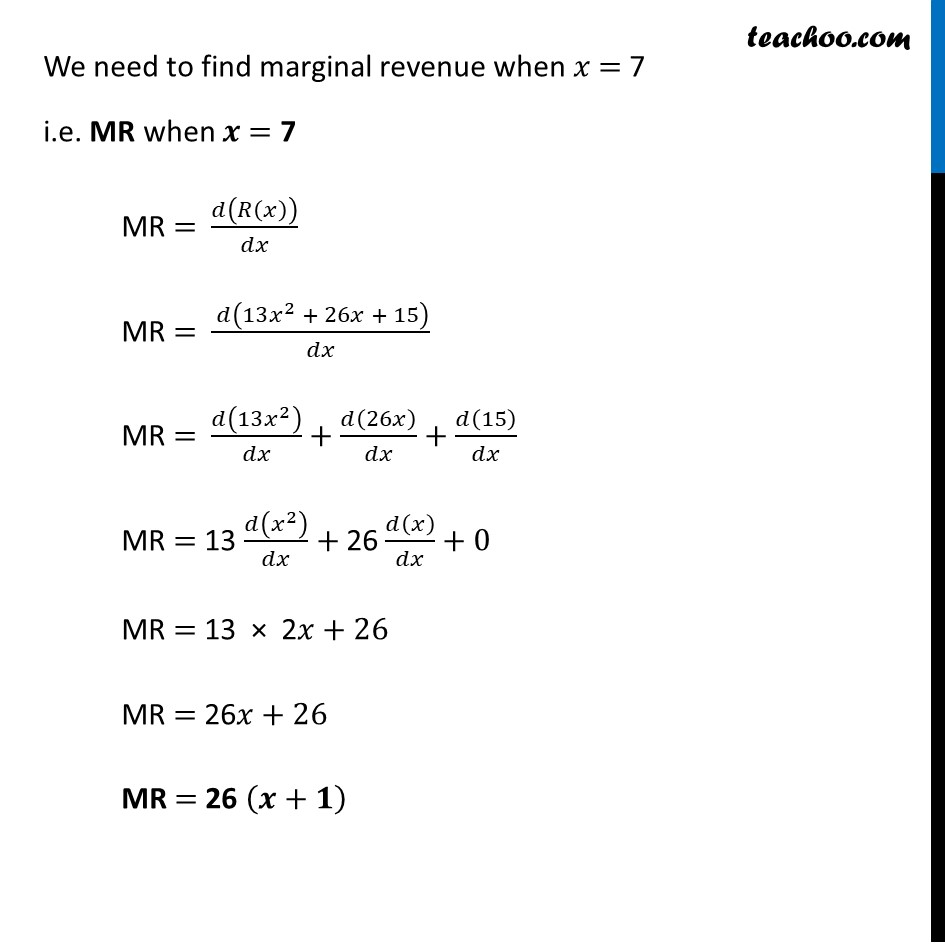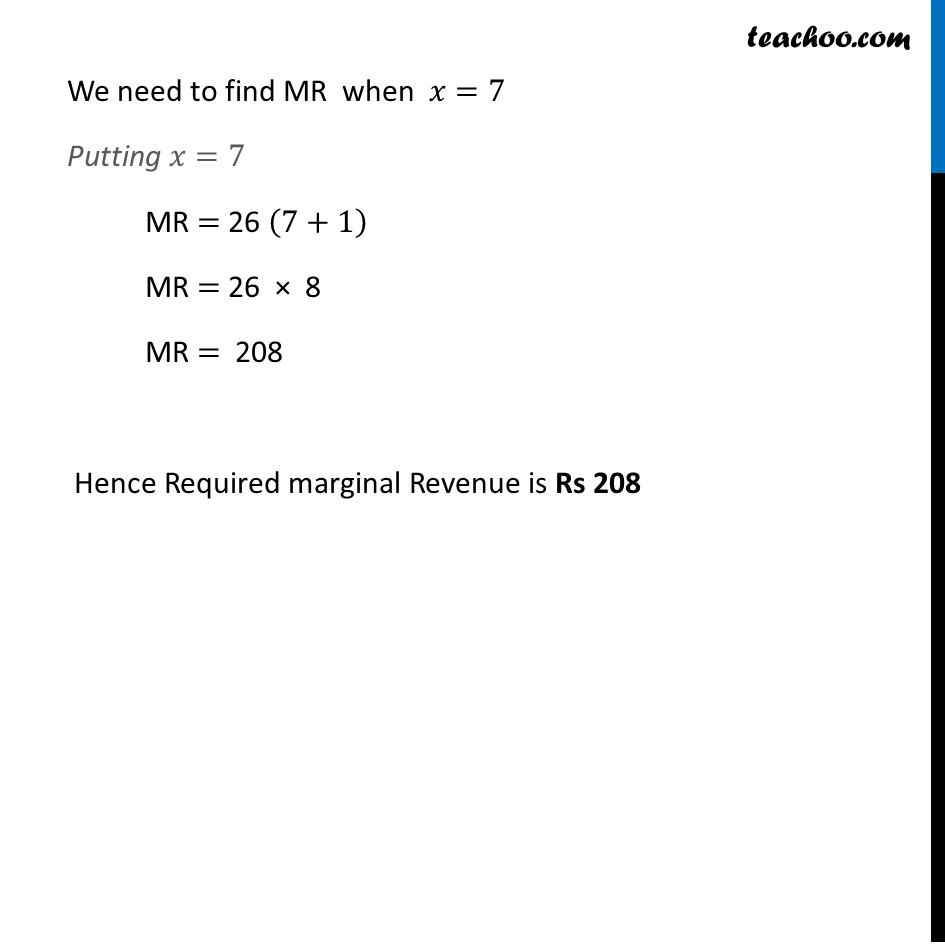Finding rate of change

Chapter 6 Class 12 Application of Derivatives (Term 1)
Concept wise### Transcript

Ex 6.1, 16 The total revenue in Rupees received from the sale of 𝑥 units of a product is given by R(𝑥) = 13𝑥2 + 26𝑥 + 15. Find the marginal revenue when 𝑥 = 7. Marginal revenue is rate of change of total revenue w. r. t the number of unit sold Let MR be marginal revenue So, MR = 𝒅𝑹/𝒅𝒙 Given, Total revenue = R(𝑥)=13𝑥^2+26𝑥+15 We need to find marginal revenue when 𝑥 = 7 i.e. MR when 𝒙 = 7 MR = 𝑑(𝑅(𝑥))/𝑑𝑥 MR = ( 𝑑(13𝑥^2 + 26𝑥 + 15))/𝑑𝑥 MR = 𝑑(13𝑥^2 )/𝑑𝑥+𝑑(26𝑥)/𝑑𝑥+𝑑(15)/𝑑𝑥 MR = 13 𝑑(𝑥^2 )/𝑑𝑥+ 26 𝑑(𝑥)/𝑑𝑥+0 MR = 13 × 2𝑥+26 MR = 26𝑥+26 MR = 26 (𝒙+𝟏) We need to find MR when 𝑥=7 Putting 𝑥=7 MR = 26 (7+1) MR = 26 × 8 MR = 208 Hence Required marginal Revenue is Rs 208